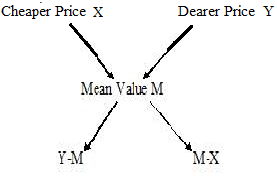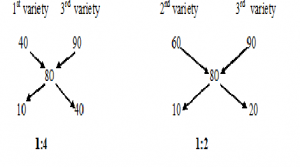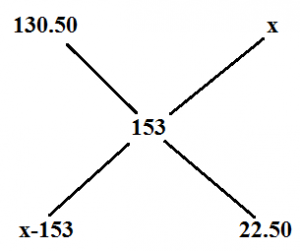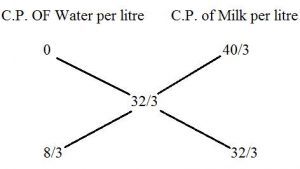# HOW TO SOLVE MIXTURE AND ALLIGATION PART 2-TIPS,TRICKS AND SHORTCUTS

Hi Bankersdaily Aspirant,

Aspirants,as you all know this is Exam Season and we had started for our Preparation.To Clear IBPS Exam we need lot of practice.Beginners find difficulty in knowing the pattern of the exam and type of questions asked in the exam.

Learning is a step by step process and you should gradually progress in that to ace the process. As you all know we have started a new Learn Series to help with your preparations for various exams like IBPS PO , IBPS RRB Scale I officer , IBPS RRB Office Assistant , IBPS Clerk , IBPS SO , SBI PO , SBI Clerk , SBI SO and many other exams in this type. Nevertheless this series will also contribute you all the tips and tricks to every miscellaneous sections and also all the important topics which are asked in the exams. If you haven’t looked at the Learn series , Please Click the below link to learn the new concepts.

## INTRODUCTION:

Hi Aspirants today we are going to discuss on a topic Mixture and Alligation. This  topic carries 1 mark in prelims and 2 mark in mains exam.

## MIXTURE:

When two or more components are mixed together then it is known as Mixture

Mixture is of two types they are:

1.Simple Mixture:When two or more different ingredients are mixed together,a simple mixture is formed

2.Compound Mixture:When two or more simple mixtures are mixed together, a compound mixture is formed.

## ALLIGATION:

It enables us to find the ratio in which two or more ingredients at the given price must be mixed to produce a mixture  of desired price.Where M=Mean Value which is between X and Y, X=Cheaper Price, Y=Price higher than the Mean Value

## BASED  ON THREE INGREDIENTS OR THREE RATIO’S:

1.The Grocer sells apples of 3 varieties whose price per kg’s are Rs.40,Rs.60  and Rs. 90 .If he sells 1kg of apple by missing all these 3 varieties for Rs.80 what is the ratio by which these varieties are to be mixed?

Explanation:1st variety 3rd variety 2nd  variety 1 4 2 1

Ratio’s by which these 3 Qualities are missed are=1:2:4

2.Tea worth Rs.126   per kg and Rs.135 per kg are mixed with a third variety in the ratio 1:1:2.If the mixture is worth Rs.153 per kg, the price of the third variety per kg will be?

Explanation:

Given that the tea worth Rs.126 and Rs.135 per kg  is mixed in the ratio 1:1.

Therefore price for 1kg=  = Rs.130.50

Now we have two price 130.50 and the price of the third variety is XThe price of the third variety=Rs.175.50

3.There are three vessels of equal capacity holds milk and water in the ratio of 1:2,2:3 and 1:4if the content of all the three vessel s are mixed in asingle vessel then.Find the ratio of milk and water in the new vessel?

Explanation:

Here there are three ratio’s

1:2=3Part

2:3=5Part

1:4=5Part

Lcm =15Litres

 Milk Water 5Litres 10Litre 6Litre 9Litre 3Litre 12Litre 14Litres 31Litre

The new Ratio is 14:31

## BASED ON REMOVING AND ADDING SOME QUANTITY :

1)How much water must be added to 60 litres of milk at 1.5 litre for Rs.20,So as to have a mixture worth Rs.10 2/3 per litre?

Explanation:

C.P for 1.5 litre of milk =Rs.20

C.P  for 1 litre of milk=Rs.40/3

C.P for 1 litre of water=Rs.0

Given Mean value=Rs.32/38/3  :   32/3= 1:4

Therefore water in the mixture in 60 litres is (1/5)*60=12 litres

2)In a 729 litres mixture of milk and water ,the ratio  of milk to water is 7:2.To get a new mixture containing milk and water in the ratio 7:3,the amount of water to be added is?

Explanation:
 729 7x 2x

9x=729           x=81

Old Mixture     New Mixture

7:2                       7:3

Already the mixture contains water in the proportion 2x  and the new mixture contains water in the proportion 3x .So x quantity of water is added  to the mixture .Therefore 81 litres of water is added to the new mixture.

3)8 litres are drawn from a cask filled with wine and is then filled with water .This operation is performed three more times.The ratio of the quantity of wine now left in the cask to that of the total solution is 16:81.How much wine does the cask originally ?

Explanation:

When the final amount of solute that is not replaced calculated as:

Initial Amount * (Volume After Removal/Volume After Replacing)^n

Final  ratio of solute not replaced to total is

Initial Ratio * (Volume After Removal/Volume After Replacing)^n

Here let us assume the initial value is 1 then Wine .Let the Quantity of wine in the cask originally be x

Therefore

1* [(x-8)^4/x]

=[16/81]

x=24litres

Wine originally in the cask is 24 Litres

4)A vessel contains mixture of liquids A and B in the ratio 3:2.When 20 litres of the mixture  is taken out and replaced by 20litres of liquid B,the ratio changes to 1:4.How many litres of liquid A was there initially present in the vessel?

Explanation:

Original ratio=3:2

3/5 * (5X-20)/5x=1/4

X=6  then the liquid A in the vessel =3X=3*6=18Litres

### IMPORTANCE OF MIXTURE AND ALLIGATION:

Mixture and Alligation is used in other Miscellaneous Topic such as

Ratio and Proportion

Time and Distance

You can reduce the time taken to solve the problem if you use Mixture and Alligation Method

YOU MAY ALSO LIKE TO READ:

TRICKS TIPS SHORTCUTS FOR ALL THE TYPES OF PROBLEMS BASED ON RACES

TIPS TRICKS AND SHORTCUT METHOD TO SOLVE BOATS AND STREAMS